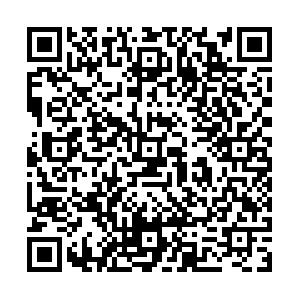# Study of the CKM angle γ sensitivity using flavor untagged ${{B^0_s}} {\rightarrow} \tilde{{D}}^{{(*)0}} {\phi}$decays• A sensitivity study on the measurement of the CKM angle $\gamma$from ${{B^0_s}}\rightarrow \tilde{D}^{(*)0}\phi$decays is conducted using the D-meson reconstructed in the quasi flavour-specific modes $K\pi$, $K3\pi$, and $K\pi{{\pi^{0}}}$, as well as CP-eigenstate modes $KK$and $\pi\pi$, where the notation $\tilde{D}^0$corresponds to a ${{D^0}}$or ${{{\kern 0.2em\overline{\kern -0.2em D}{}}{}^0}}$meson. The LHCb experiment is presented as a use case. A statistical uncertainty of approximately $8^{\circ}-19^{\circ}$can be achieved with the $pp$collision data collected in the LHCb experiment from 2011 to 2018. The sensitivity to $\gamma$should be of the order $3^{\circ}-8^{\circ}$after accumulating 23 fb-1 of $pp$collision data by 2025, whereas it is expected to improve further by 300 fb-1 by the second half of the 2030 decade. The accuracy is dependent on the strong parameters ${{r^{(*)}_{B}}}$and ${{\delta^{(*)}_{B}}}$, which together with $\gamma$describe the interference between the leading amplitudes of the ${{B^0_s}}\rightarrow \tilde{D}^{(*)0}\phi$decays.
••Get Citation
D. Ao, D. Decamp, W. B. Qian, S. Ricciardi, H. Sazak, S. T’Jampens, V. Tisserand, Z. R. Wang, Z. W. Yang, S. N. Zhang and X. K. Zhou. Study of the CKM angle γ sensitivity using flavor untagged ${{B^0_s}}\rightarrow \tilde{D}^{(*)0}\phi$decays[J]. Chinese Physics C. doi: 10.1088/1674-1137/abd16d
D. Ao, D. Decamp, W. B. Qian, S. Ricciardi, H. Sazak, S. T’Jampens, V. Tisserand, Z. R. Wang, Z. W. Yang, S. N. Zhang and X. K. Zhou. Study of the CKM angle γ sensitivity using flavor untagged ${{B^0_s}}\rightarrow \tilde{D}^{(*)0}\phi$decays[J]. Chinese Physics C.Milestone
Revised: 2020-11-03
Article Metric

Article Views(26)
Cited by(0)
Policy on re-use
To reuse of Open Access content published by CPC, for content published under the terms of the Creative Commons Attribution 3.0 license (“CC CY”), the users don’t need to request permission to copy, distribute and display the final published version of the article and to create derivative works, subject to appropriate attribution.
###### 通讯作者: 陈斌, bchen63@163.com
• 1.

沈阳化工大学材料科学与工程学院 沈阳 110142

Title:
Email:

## Study of the CKM angle γ sensitivity using flavor untagged ${{B^0_s}} {\rightarrow} \tilde{{D}}^{{(*)0}} {\phi}$decays

• 1. University of Chinese Academy of Sciences, Beijing 100049, China
• 2. Univ. Grenoble Alpes, Univ. Savoie Mont Blanc, CNRS, IN2P3-LAPP, Annecy, France
• 3. STFC Rutherford Appleton Laboratory, Didcot, United Kingdom
• 4. Université Clermont Auvergne, CNRS/IN2P3, LPC, Clermont-Ferrand, France
• 5. Center for High Energy Physics, Tsinghua University, Beijing 100084, China
• 6. School of Physics State Key Laboratory of Nuclear Physics and Technology, Peking University, Beijing 100871, China

Abstract: A sensitivity study on the measurement of the CKM angle $\gamma$from ${{B^0_s}}\rightarrow \tilde{D}^{(*)0}\phi$decays is conducted using the D-meson reconstructed in the quasi flavour-specific modes $K\pi$, $K3\pi$, and $K\pi{{\pi^{0}}}$, as well as CP-eigenstate modes $KK$and $\pi\pi$, where the notation $\tilde{D}^0$corresponds to a ${{D^0}}$or ${{{\kern 0.2em\overline{\kern -0.2em D}{}}{}^0}}$meson. The LHCb experiment is presented as a use case. A statistical uncertainty of approximately $8^{\circ}-19^{\circ}$can be achieved with the $pp$collision data collected in the LHCb experiment from 2011 to 2018. The sensitivity to $\gamma$should be of the order $3^{\circ}-8^{\circ}$after accumulating 23 fb-1 of $pp$collision data by 2025, whereas it is expected to improve further by 300 fb-1 by the second half of the 2030 decade. The accuracy is dependent on the strong parameters ${{r^{(*)}_{B}}}$and ${{\delta^{(*)}_{B}}}$, which together with $\gamma$describe the interference between the leading amplitudes of the ${{B^0_s}}\rightarrow \tilde{D}^{(*)0}\phi$decays.

### HTMLI.   INTRODUCTIONII.   FORMALISMIII.   EXPECTED YIELDSIV.   SENSITIVITY STUDY FOR RUN 1 & 2 LHCB DATASETV.   PROSPECTIVE ON SENSITIVITY TO $\gamma$ FOR RUNS $1-3$ AND FULL HIGH-LUMINOSITY LHC (HL-LHC) LHCb DATASETS
PDF查看关注分享

Top

### 目录/DownLoad:  Full-Size Img  PowerPoint